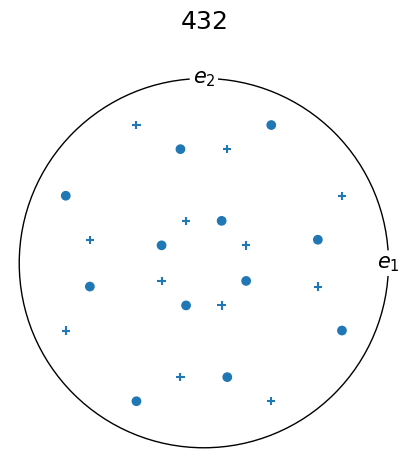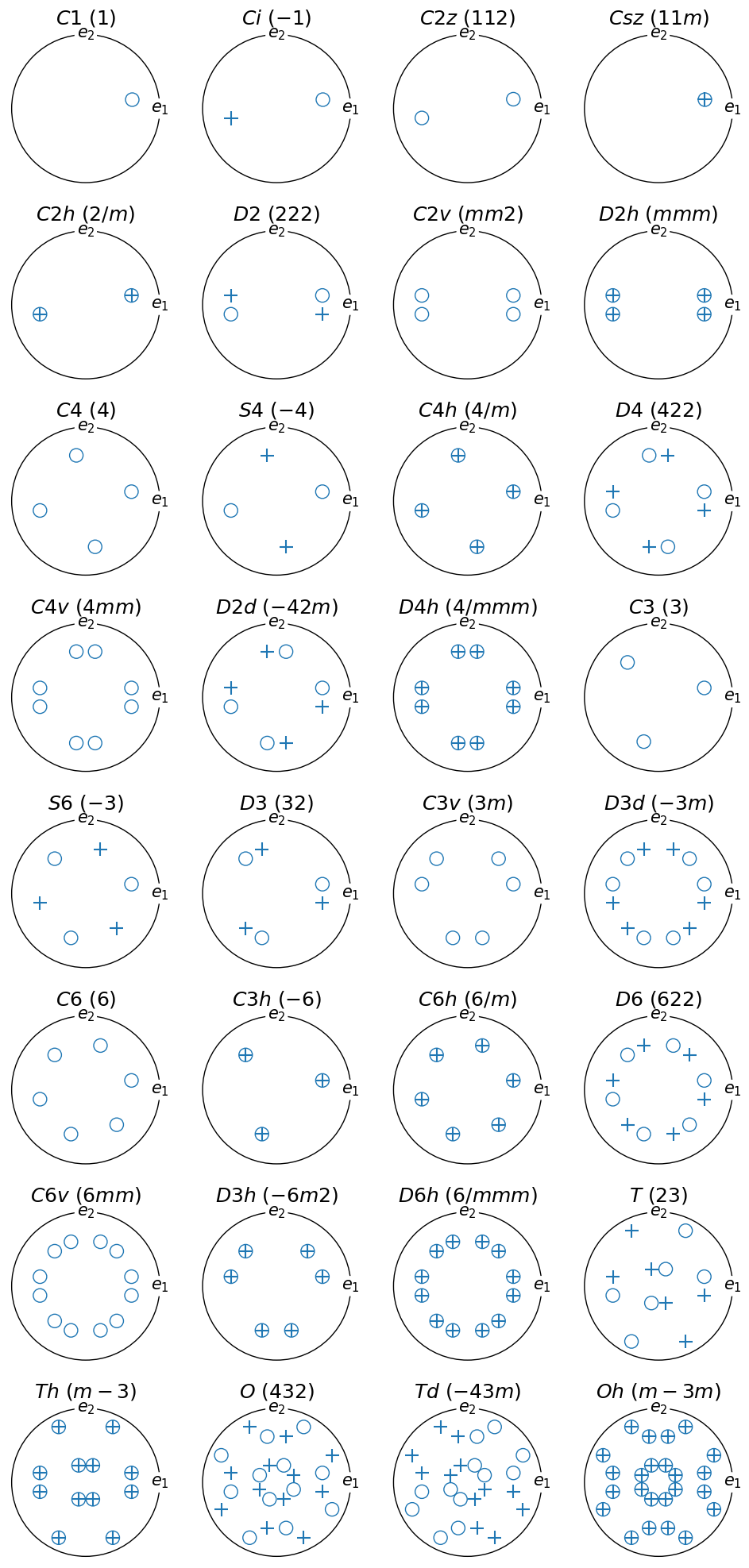# Visualizing point groups#

This tutorial shows point group symmetry operations in the stereographic projection.

Vectors located on the upper (z >= 0) hemisphere are displayed as points (o), whereas vectors on the lower hemisphere are reprojected onto the upper hemisphere and shown as crosses (+) by default. For more information about plot formatting and visualization, see Vector3d.scatter().

Further explanation of these figures is provided at http://xrayweb.chem.ou.edu/notes/symmetry.html#point.

:

%matplotlib inline
from matplotlib import pyplot as plt
import numpy as np

from orix import plot
from orix.quaternion import Rotation, symmetry
from orix.vector import Vector3d

plt.rcParams.update({"font.size": 15})


For example, the O (432) point group:

:

symmetry.O.plot()$$(\mathbf{e_1}, \mathbf{e_2}, \mathbf{e_3})$$ are the unit vectors of the standard Cartesian (orthonormal) reference frame (see the crystal reference frame tutorial for more details).

The stereographic projection of all point groups is shown below:

:

# fmt: off
schoenflies = [
"C1", "Ci",                                          # triclinic,
"C2x", "C2y", "C2z", "Csx", "Csy", "Csz", "C2h",     # monoclinic
"D2", "C2v", "D2h",                                  # orthorhombic
"C4", "S4", "C4h", "D4", "C4v", "D2d", "D4h",        # tetragonal
"C3", "S6", "D3x", "D3y", "D3", "C3v", "D3d", "C6",  # trigonal
"C3h", "C6h", "D6", "C6v", "D3h", "D6h",             # hexagonal
"T", "Th", "O", "Td", "Oh",                          # cubic
]
# fmt: on

assert len(symmetry._groups) == len(schoenflies)

schoenflies = [
s for s in schoenflies if not (s.endswith("x") or s.endswith("y"))
]

assert len(schoenflies) == 32

orientation = Rotation.from_axes_angles((-1, 8, 1), 65, degrees=True)

fig, ax = plt.subplots(
nrows=8,
ncols=4,
figsize=(10, 20),
subplot_kw=dict(projection="stereographic"),
)
ax = ax.ravel()

for i, s in enumerate(schoenflies):
sym = getattr(symmetry, s)

ori_sym = sym.outer(orientation)
v = ori_sym * Vector3d.zvector()

# reflection in the projection plane (x-y) is performed internally in
# Symmetry.plot() or when using the reproject=True argument for
# Vector3d.scatter()
v_reproject = Vector3d(v.data.copy())
v_reproject.z *= -1

# the Symmetry marker formatting for vectors on the upper and lower
# hemisphere can be set using kwargs and reproject_scatter_kwargs,
# respectively, for Symmetry.plot()

# vectors on the upper hemisphere are shown as open circles
ax[i].scatter(v, marker="o", fc="None", ec="k", s=150)
# vectors on the lower hemisphere are reprojected onto the upper
# hemisphere and shown as crosses
ax[i].scatter(v_reproject, marker="+", ec="C0", s=150)

ax[i].set_title(f"${s}$ $({sym.name})$")
ax[i].set_labels("$e_1$", "$e_2$", None)

fig.tight_layout()